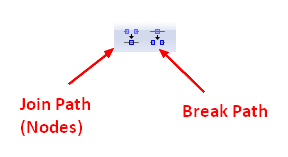# SVG - Path

Image Vector - Path in Svg.

Path is the generic element to define a shape.

Complex shapes composed only of straight lines can be created as polylines.

## Syntax

``````<!-- A triangle -->
<path
d="M 10,150 L 70,10 L 130,150 z"
fill="#D5D8CB"
stroke="#ECDCC6"
stroke-width="6"/>```
```

### d attribute

The shape of a path element is defined by one attribute: d that contains a suite of command.

All of the commands also come in two variants.

• An uppercase letter specifies absolute coordinates on the page,
• and a lowercase letter specifies relative coordinates (e.g. move from the last point 10px up and 7px to the left).

Coordinates in the “d” attribute are always unitless and hence in the user coordinate system.

### Style attributes

You can apply also style attribute such as:

• fill (none, transparent)
• stroke
• stroke-width

## Commands

### M - Move to

No line is drawn.

• M 10 10 to move to (10,10). The “Move to” command is called with the letter M.
• m dx dy to move to the relatif position (dx, dy).
``````<svg >

<path d="M 10 10 m 40 0"/>

<!-- Equivalent to Points -->
<circle cx="10" cy="10" r="5" />
<circle cx="50" cy="10" r="5" />

</svg>```
```
``````path {
fill: none;
stroke: #000;
stroke-width: 1.5px;
}
circle {
fill: red;
}```
```

### Draw a straight line

#### L - Line To

L takes two parameters—x and y coordinates—and draws a straight line from the current position to a new position.

• L x y to draw a line with absolute coordinates
• l dx dy to draw a line with relatif coordinates
``````<svg width="200" height="30" xmlns="http://www.w3.org/2000/svg">

<path d="M 10 10 L 30 10"/>
<!-- Points -->
<circle cx="10" cy="10" r="2"/>
<circle cx="30" cy="10" r="2"/>

</svg>```
```
``````path {
fill: none;
stroke: #000;
stroke-width: 1.5px;
}
circle {
fill: red;
}```
```

#### H - Draws a horizontal line

The commands only take one argument since it move only in one direction.

• H x to move absolutely
• h dx to move relatively
``````<svg width="200" height="30" xmlns="http://www.w3.org/2000/svg">

<path d="M 10 10 H 30"/>
<!-- Points -->
<circle cx="10" cy="10" r="2"/>
<circle cx="30" cy="10" r="2"/>

</svg>```
```
``````path {
fill: none;
stroke: #000;
stroke-width: 1.5px;
}
circle {
fill: red;
}```
```

#### V - Draws a vertical line.

The commands only take one argument since it move only in one direction.

• V y to move absolutely
• v dy to move relatively
``````<svg width="200" height="40" xmlns="http://www.w3.org/2000/svg">

<path d="M 10 10 V 30"/>
<!-- Points -->
<circle cx="10" cy="10" r="2"/>
<circle cx="10" cy="30" r="2"/>

</svg>```
```
``````path {
fill: none;
stroke: #000;
stroke-width: 1.5px;
}
circle {
fill: red;
}```
```

### Z- Close the path

This command draws a straight line from the current position back to the first point of the path. There is no difference between the uppercase and lowercase command. Z or z

``````<svg width="200" height="40" xmlns="http://www.w3.org/2000/svg">

<path d="M 10 10 V 30 H 30 z"/>
<!-- Points -->
<circle cx="10" cy="10" r="2"/>
<circle cx="10" cy="30" r="2"/>
<circle cx="30" cy="30" r="2"/>

</svg>```
```
``````path {
fill: none;
stroke: #000;
stroke-width: 1.5px;
}
circle {
fill: red;
}```
```

## Javascript Path Generation

``````data=[
{x: 20, y: "20"}
, {x: 3, y: "3"}
]

let d = `
M\${data.x} \${data.y}
\${data.slice(1).map(d => {
return `L\${d.x} \${d.y}`;
}).join(' ')}
`;
console.log(d)```
```

## Documentation / Reference

Discover MoreInkscape - Path

in Inkscape Paths are arbitrary shaped objects created from: nodes and segment. A shapes has a defined structure whereas a path is a series of joined point coordinates. A rectangle will always keep...SVG - (Straight) Line

You can draw a straight line in SVG with the following two elements: The line element defines a line segment that starts at one point and ends at another. See Path - Draw a straight...SVG - Bezier Curve

A path can be describe as a series of Bezier curves There are an infinite number of Bezier curves, but only two simple ones are available in path elements: a cubic one, called with C, and a quadratic...SVG - Curve (Line)

in SVG. A curved line is just designed with a path element. There are three different path commands that you can use to create smooth curves. bezier curve, an “arc” or part of a circle....SVG - Element

svg element are elements of the SVG language They defines: geometric shape such as circle or the more generic path element Bounded together below the svg root element, they define a vectoriel image...SVG - Marker (arrowheads or polymarkers)

The element defines the graphics that is to be used for drawing: arrowheads or polymarkers on a given path, line, polyline or polygon element. Element/marker...SVG - Rectangle (rect)

To draw a rectangle, you can use: * the special shortcut element rect. See * the path element. See The rect element without the fill attribute is black. The rect element with the path...SVG - Shape and Path

The representation of geometric object (shape) in svg. They are in the grammar. You need to use the path element to create themSVG - TextPath element

on a path